# Mixed References in Excel - a Beginner's GuideAgnes Lo

## What is a mixed reference?

Mixed reference Excel definition: A mixed reference is made up of both an absolute reference and relative reference. This means that part of the reference is fixed, either the row or the column, and the other part is relative.

An absolute reference refers to a fixed point of reference where the return value always remains the same even if the cell or formula moves. A relative reference refers to a changing point of reference, where the return value changes depending on where the cell or formula moves to. For more detail and examples of each, check out our resources on absolute references and relative references

Since a mixed cell reference includes an absolute reference, a \$ (dollar sign) is present in the formula. There is only one dollar sign present to hold either the row or column constant, instead of two dollar signs in the usual absolute reference set up.

A mixed reference comes in these two common forms:

1. =\$A1 (\$ in front of column, holding the column constant)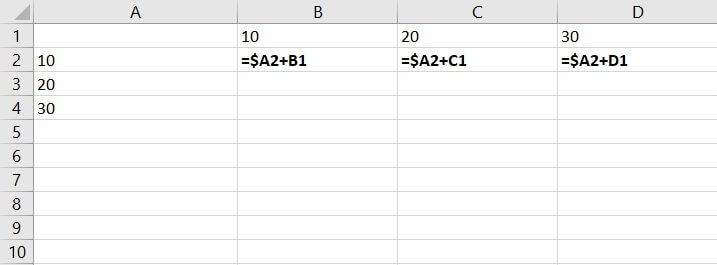1. =A\$1 (\$ in front of row, holding the row constant)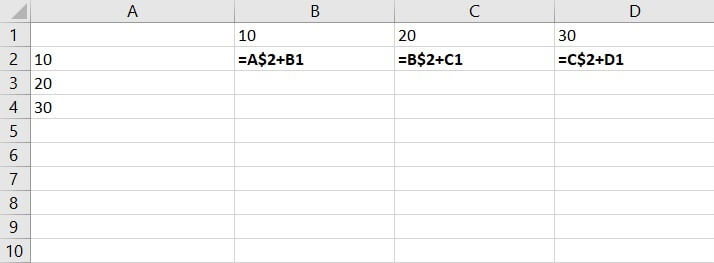Mixed references are frequently employed in Excel as they are quite handy in situations where the same calculations are used across rows and columns.

They are slightly more difficult to begin with compared to absolute references and relative references; nonetheless, they help to save time and reduce errors.

## How to make a mixed reference in Excel

Use this free Excel file to practice mixed references along with the tutorial.

Here are the steps on how to make a basic mixed cell reference in Excel.

1. Choose a cell where you would like to create a mixed reference. Cell B2 in this example.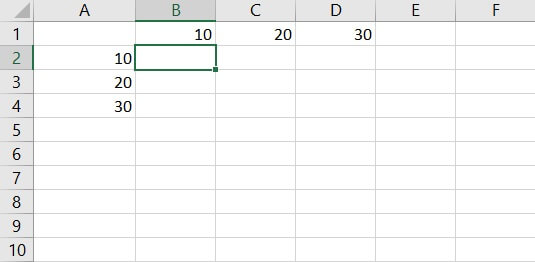1.  Assuming the idea is to sum up the numbers, in the formula of B2 enter “=” (the equal sign) then select the point of reference – Cell A2 and B1.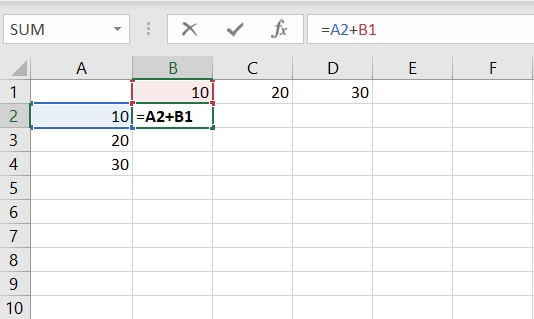1. Hold the column and row constant:

a. To hold the column constant, add a \$ (dollar sign) in front of A, then copy the formulas across the table.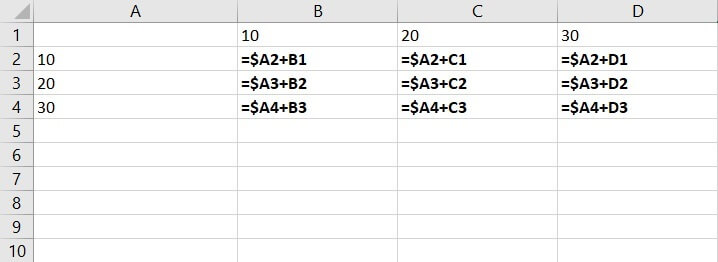The results are below: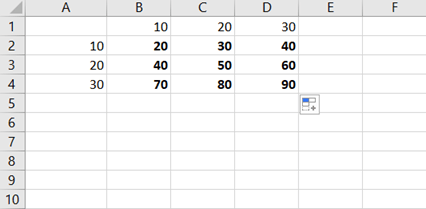b. To hold the row constant, add a \$ (dollar sign) in front of row instead (=A\$2+B1), then copy the formulas across the table.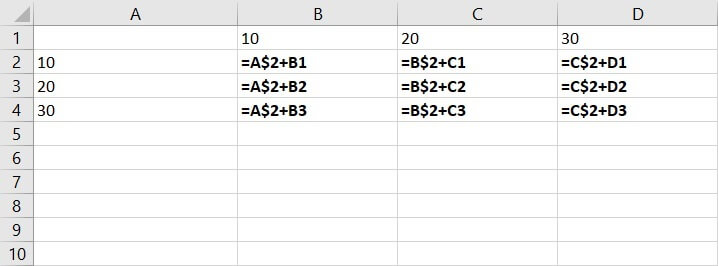The results are below: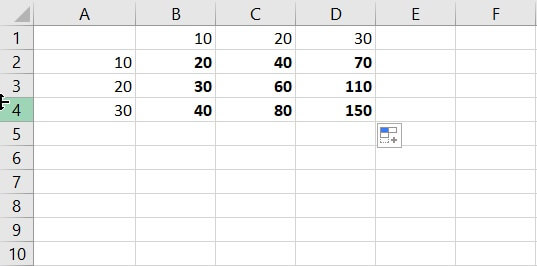As seen from those two examples above, holding the column or row constant produces very different results. Before assigning the dollar sign to a column or row, it’s recommended to review the calculations and logic on hand to avoid errors.

## Examples of mixed reference

Versatile and convenient, mixed cell references are frequently used in Excel spreadsheets. They can be used with both numbers and text.

Mixed reference is particularly preferred when doing calculations in Excel, as it helps users to evaluate figures across scenarios and conditions. It saves time by copying the formulas across the Excel table as well.

Below is an example of the application of mixed reference:

The company secretary has been asked to calculate the annual income tax for several top executives in the company. She has been given their annual income between 2017 and 2019 and their respective tax rate.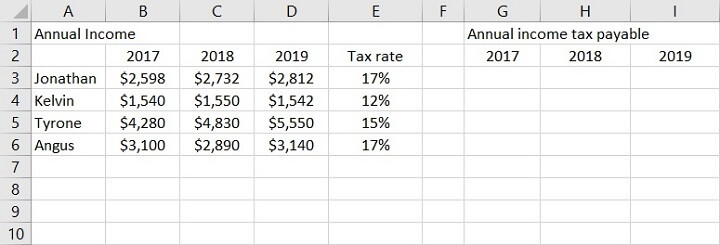To find out their respective annual income tax payable, the calculation is = annual income * tax rate

• In Jonathan’s case, it would be = 2598*17%
• In Excel expression, it would be =B3*E3.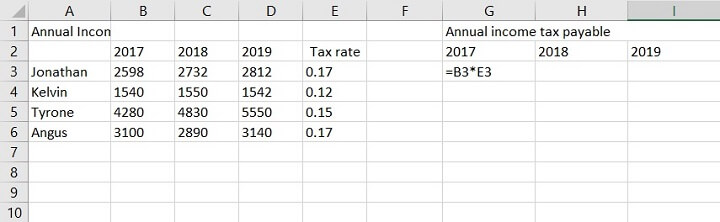The return value is \$441.66.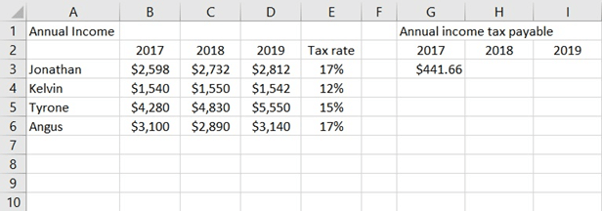To calculate the rest, since the tax rate is always in column E, column E is to be held constant in the calculation.

To do so, add \$ to the calculation =B3*\$E3: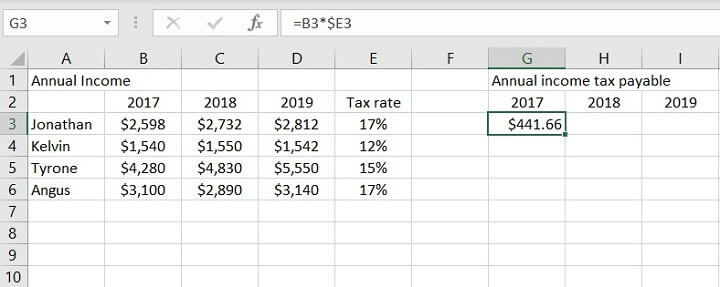Then, copy the formula across the rest of the remaining cells.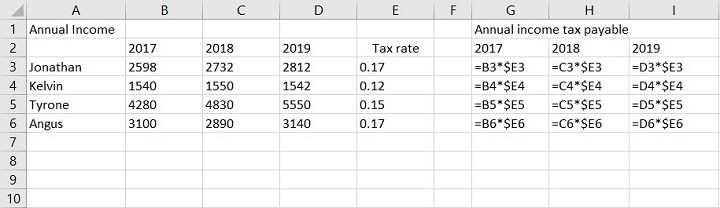The return values are below: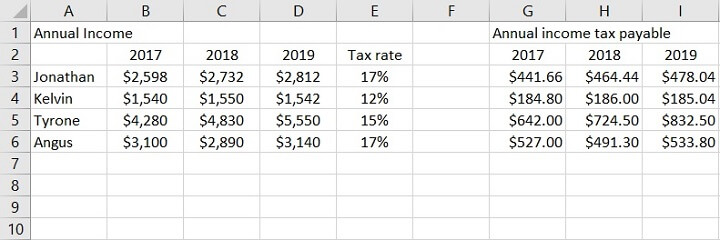If a mixed reference was not applied in the scenario above, and only a relative reference used, the calculations would not be correct.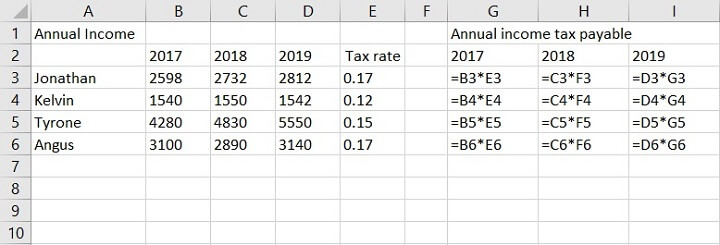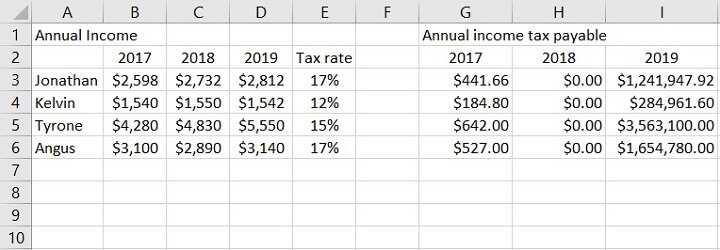In this example, the data sets are all variables, but in particular, the tax rate holds constant for each tax player across the years.

As tax rate is available under column E only, when applying the formulas to other cells, it’s important to hold column E constant to produce the correct value.

## Summary

Mixed reference in Excel means only part of the reference is fixed, either the row or the column, and the other part is relative. Unlike absolute references, only one \$ (dollar sign) is applied, either in front of the column or row number.

Given its elaborate nature, mixed references can compute complicated situations and scenarios easily and are often used to create dashboards and forecasts. Users can copy and paste the formula in the table to minimize manual calculations and errors.

It’s essential to review calculations and logic on hand before applying mixed references as locking the column or row will produce dramatically different results.

To learn more about working with references and other awesome tools in Excel try our Excel - Basic and Advanced course today. Or you can explore our free Excel in an Hour crash course to cover some Excel basics.